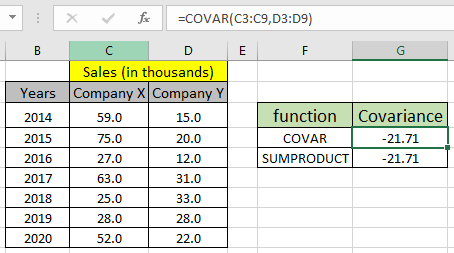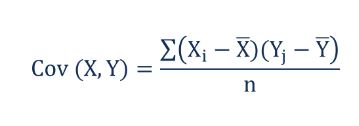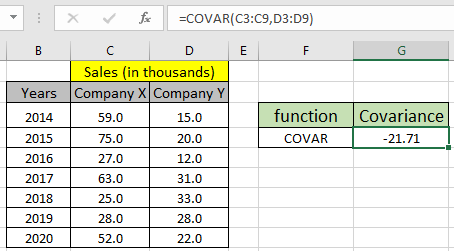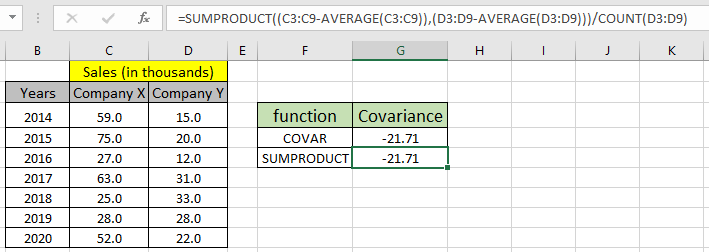# How to use the COVAR function in ExcelIn this article, we will learn How to use the COVAR function in Excel.

What is covariance between two datasets?

The measurement of directional relation between the two datasets can be compared using the covariance function. Covariance value for the given datasets can be positive, negative or zero. Positive covariance denoted that the two given datasets move in the same direction i.e. positive change in one dataset leads to the positive change in the other dataset whereas negative covariance denoted that the two datasets are move inversely or opposite direction i.e. positive change in one data set leads to the negative change in the other dataset. Zero covariance means that the two datasets do not depend on each other. If you want to know how much dependency in between the two relational datasets can be calculated using the correlation coefficient. Generally in statistics, calculating the covariance between the two datasets (X, Y) using the formula shown below.Here X and Y are two different datasets with X and Ybe the respective mean for the datasets. And i denotes the individual values of the corresponding datasets. N is the number of values in the dataset (n must be equal for the both datasets). Learn about how to calculate the covariance using the COVAR function in excel.

COVAR function in Excel

COVAR function takes the two equal lenth arrays as arguments and returns the relational covariance between the two arrays.

COVAR function syntax:

 =COVAR(array_1, array_2)

array_1 : First dataset values given as array.

array_2 : Second dataset values given as array.

Example :

All of these might be confusing to understand. Let's take an example to understand how to use theCOVAR function to get covariance value. Here we have sales of the two companies X and Y with year wise datas. Manager of a company suspects there is a relation between the two companies' sales values. So he ran the below formula on the dataset.

Use the formula:

 = COVAR (C3:C9, D3:D9)The formula returns -21.71 as covariance which means that the both company's sales are inversely directional. Knowing the Covariance formula for the given dataset we can calculate the covariance using the SUMPRODUCT function and AVERAGE function

Use the formula :

 =SUMPRODUCT((C3:C9-AVERAGE(C3:C9)),(D3:D9-AVERAGE(D3:D9)))/COUNT(D3:D9)The SUMPRODUCT formula returns the same result as COVAR function. This formula doesn't operate, if any argument to the formula is non-numeric whereas COVAR function just ignores the value and returns the COVARIANCE for the remaining datasets.

Use the new covariance function in Excel for two different purposes.

1. Use COVARIANCE.P for population dataset.
2. Use COVARIANCE.S for sample dataset.

Here are some observational notes using the COVAR function as shown below.

Notes :

1. The function works with numbers only.
2. Both argument arrays must be the same size else the function returns #NA error.
3. If any argument in the COVAR function is non-numeric, the function ignores the value and returns the covariance for the remaining datasets.
4. The SUMPRODUCT function returns #VALUE! Error, if any argument to the sumproduct formula is non-numeric.
5. Operators like equals to ( = ), less than equal to ( <= ), greater than ( > ) or not equals to ( <> ) can be performed within a formula applied, with numbers only.

Hope this article about How to use the COVAR function in Excel is explanatory. Find more articles on statistical formulas and related Excel functions here. If you liked our blogs, share it with your friends on Facebook. And also you can follow us on Twitter and Facebook. We would love to hear from you, do let us know how we can improve, complement or innovate our work and make it better for you. Write us at info@exceltip.com

Related Articles :

How to Find Correlation Coefficient in Excel : The correlation coefficient of a data set is a statistical number that tells how strongly two variables are related to each other. Learn about mathematical formula and relational Correlation function here

How to use the SUMPRODUCT function in Excel : Returns the SUM after multiplication of values in multiple arrays using the SUMPRODUCT in excel.

How to use the AVERAGE function in Excel : AVERAGE function in Excel takes a data sample and returns the mean or average fro the input values in Excel.

How to use the VAR function in Excel : Calculate the variance for the sample data numbers in excel using the VAR function in Excel.

Regressions Analysis in Excel : Regression is an Analysis Tool, which we use for analyzing large amounts of data and making forecasts and predictions in Microsoft Excel.

How to Create Standard Deviation Graph : The standard deviation graph tells how much the data is clustered around the mean of the data. Learn how to plot the standard deviation graph in Excel.

How to use the STDEV Function in Excel : Calculate the standard deviation for the data numbers in excel using the STDEV function in Excel.

Popular Articles :

How to use the VLOOKUP Function in Excel : This is one of the most used and popular functions of excel that is used to lookup value from different ranges and sheets.

How to use the IF Function in Excel : The IF statement in Excel checks the condition and returns a specific value if the condition is TRUE or returns another specific value if FALSE.

How to use the SUMIF Function in Excel : This is another dashboard essential function. This helps you sum up values on specific conditions.

How to use the COUNTIF Function in Excel : Count values with conditions using this amazing function. You don't need to filter your data to count specific values. Countif function is essential to prepare your dashboard.

Terms and Conditions of use

The applications/code on this site are distributed as is and without warranties or liability. In no event shall the owner of the copyrights, or the authors of the applications/code be liable for any loss of profit, any problems or any damage resulting from the use or evaluation of the applications/code.